# Using Geo-coordinates in Alteryx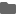2021.01.22

(日本語はこちらから)

## Basic Info

Latitude and Longitude information in the data is very important in creating a map in Alteryx.

The longitude values range from -180 to +180 depending on the location. Negative values represent the Western hemisphere, while positive values represent the Eastern hemisphere, with zero degree longitude serving as the prime meridian. At the equator, one unit of longitude covers about 111 km of distance. This distance decreases as we approach towards the poles.

Similarly latitude values range from -90 to +90 depending on the location. Negative values represent the Northern hemisphere, while positive values represent the Southern hemisphere, with zero degree latitude serving as the equator. One unit of latitude covers about 111 km distance approximately anywhere on the earth.

For overall understanding, see below: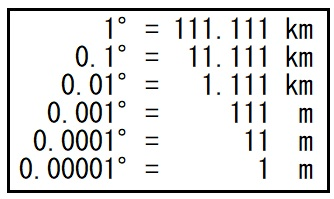In general, 5 digits of precision after the decimal point is sufficient enough. Five decimal places are accurate up to 1.1 meter and useful for general mapping needs. For example, when searching for entire buildings based on a trade area, 5 decimals should do the trick.

Let us take a working example of Tokyo Tower whose precise coordinates are (35.65859139654237, 139.74543558220884). If we start by rounding off the numbers to nearest digit we can narrow down to a range of 111.11 km diameter with the coordinates (36, 140) as shown below: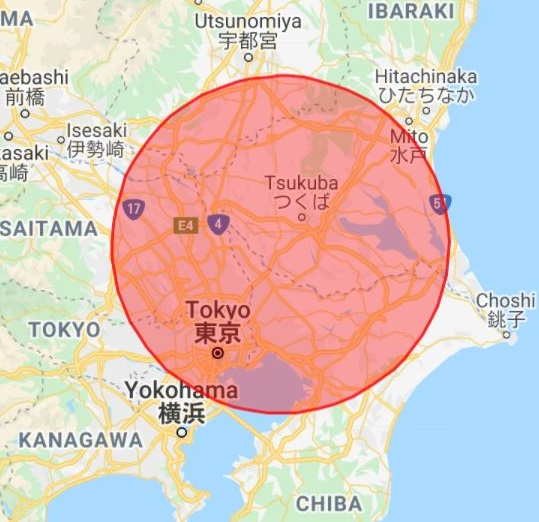If we round the coordinates to one decimal we get (35.7, 139.7) which is an area covering 11.1 km as shown below: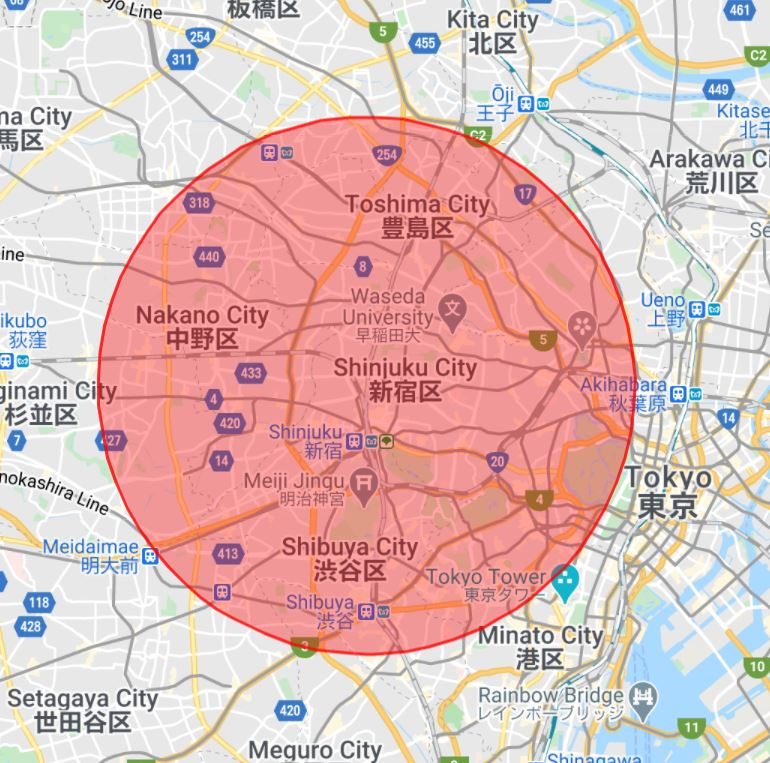Zooming further if we round the coordinates to two decimals we get (35.66, 139.74) which is an area covering 1.1 km as shown below: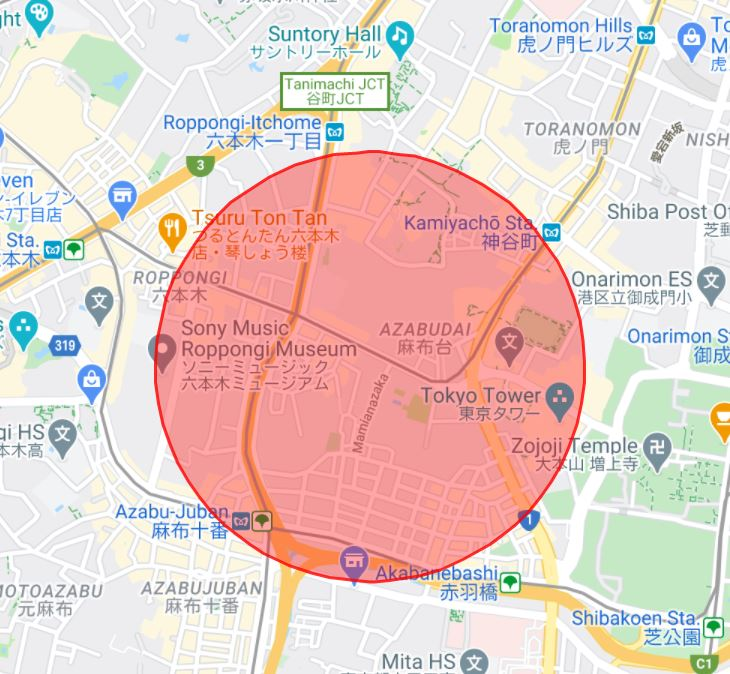With three decimal precision, new coordinates are (35.659, 139.745) and cover 111 m area which is pretty close to Tokyo tower as shown below: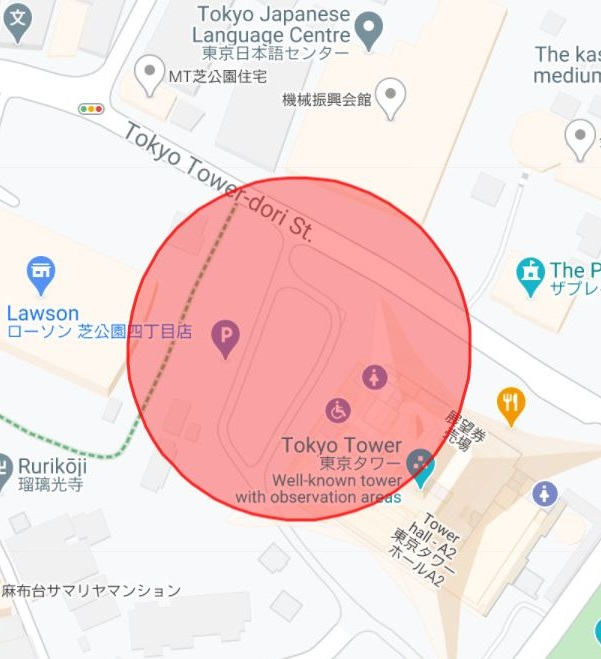With four decimal precision, new coordinates are (35.6586, 139.7454) and cover 11 m area which is within the Tokyo tower’s limit as shown below: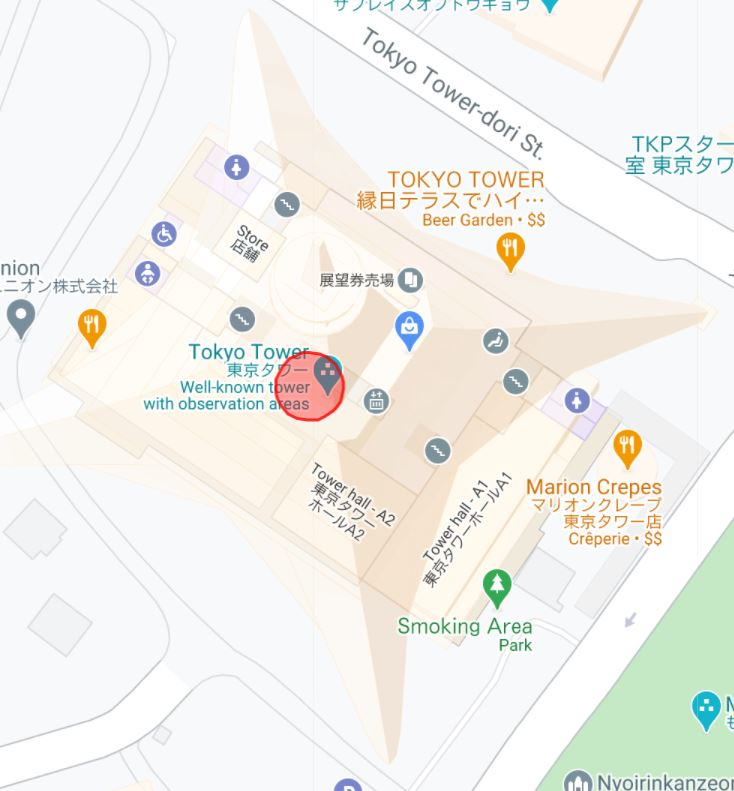Finally with five decimal precision, new coordinates are (35.65859, 139.74536) and cover 1.1 m area which is very close to the actual centroid of the Tokyo tower as shown below: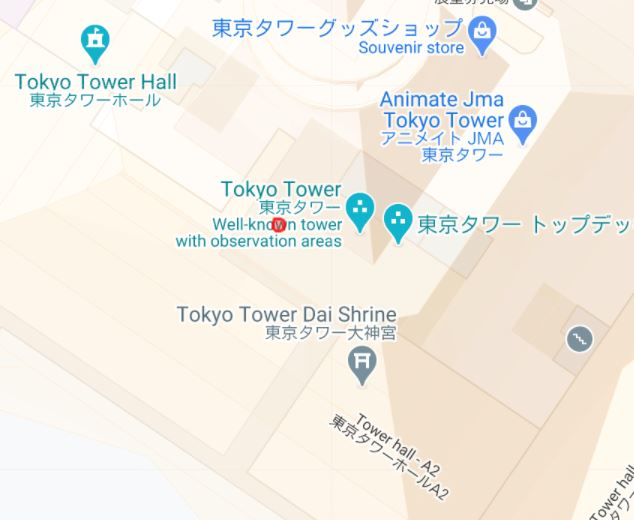Thus having five decimal places in the latitude and longitude gives a fair understanding of various places on a map.

## Summary

This post gives a basic overview on the latitude and longitude system used for creating maps.

### Alteryxの導入なら、クラスメソッドにおまかせください